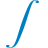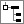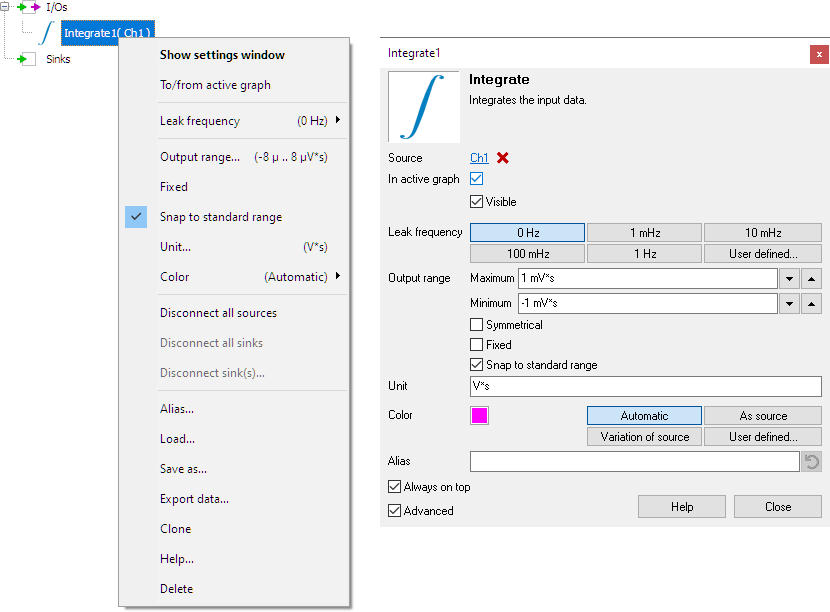# IntegrateThe Integrate I/O integrates the source's data, it is the inverse of the Differentiate operation.

A typical application of the Integrate I/O is when measuring acceleration sensor signals. Integrating the acceleration signal will give a signal representing the speed. Integration of the speed will give the relative position.

## Properties

To control the behavior of the Integrate I/O, several properties are available. These can be accessed through a popup menu which is shown when the I/O is right clicked in the Object Tree. The properties can also be accessed through its settings window which is shown when the I/O is double clicked in the Object Tree. To open the Object tree, click theShow object tree button.By default, the settings window only shows the most used settings. When Advanced is ticked, the extended window with all settings is shown. See also the program settings.

### Leak frequency

An ideal integrator integrates all frequencies in a signal. In practice, unwanted offsets can cause problems with an ideal integrator. Therefore the integrate I/O contains a leakage parameter, controlled by the Leak frequency property. All frequencies below the leak frequency will be suppressed.

### Related information

#### Gain / Offset

The Gain / Offset I/O multiplies a signal with a constant gain factor and adds a constant offset.

The Add / Subtract I/O adds or subtracts data of two or more sources.

#### Multiply / Divide

The Multiply / Divide I/O multiplies or divides data of two ore more sources.

#### Sqrt

The SQRT I/O calculates the square root of each sample of the source's data.

#### ABS

The ABS I/O takes the absolute value of each sample of the source's data.

#### Differentiate

The Differentiate I/O differentiates the source's data.

#### Logarithm

The Logarithm I/O calculates the logarithm of the source's data.